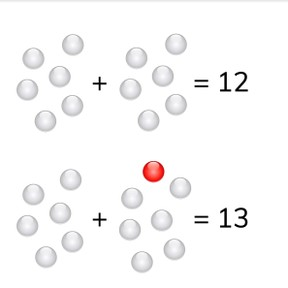Addition to 20 with doubles and near doubles

# Addition to 20 with doubles and near doubles8,000 schools use Gynzy92,000 teachers use Gynzy1,600,000 students use Gynzy

## General

Students learn to use doubles and near doubles to calculate totals to 20.

-1.OA.C

## Relevance

If you are able to calculate doubles, you can also use near doubles to find totals. You can calculate easier this way.

## Introduction

The interactive whiteboard shows a few addition problems with doubles. The answers are all jumbled. The students must determine which answer belongs to each addition problem and drag that number to the addition problem.

## Development

Explain to students that an addition problem with two numbers that are near a double can be calculated using the double. The image with white beads and one red bead on the board help to visualize this process. Explain that when you know what the total of a doubled number is, you can use that number to determine the answer of the near double, that is one more and one less. If the addend after the plus sign is one less than the double, then the answer is also one less. If the addend after the plus sign is one more than the double, then the answer is also one more. Complete a few addition problems with the students, checking the arrows which indicate if the number is one more or one less. In the final problems, a few numbers are missing, and students must determine what these missing numbers are. You can erase the grey boxes to reveal the answers.

To check that students understand using doubles and near doubles to add to 20, you can ask the following questions:
- What do you do if the addition problem is one less?
- What do you do if the addition problem has one more?

## Guided practice

Students first practice calculating near doubles where they only need to calculate one more. Then they practice with near doubles where they only need to calculate one less. Finally they practice with a near double where they are asked to calculate one more and one less.

## Closing

Repeat the goal and ask students why it is useful to be able to add near doubles. Give each student a number card with a number from one to ten. The students walk around and find their double. When they have found their double, they calculate their total. They must then also calculate their total with one more, and one less, so their near doubles. They may then get a new number card from the teacher and repeat the process.

## Teaching tips

If students have difficulty with this goal, they can make use of blocks or manipulatives to help support the addition process. Students must be comfortable with adding doubles before they are able to quickly work with near doubles.

## Instruction materials

Number cards to ten, blocks or manipulatives

### The online teaching platform for interactive whiteboards and displays in schools

• Save time building lessons

• Manage the classroom more efficiently

• Increase student engagement